mobile_mobilenet - CSDN

• 神经网络学习小记录23——MobileNet模型的复现详解学习前言什么是MobileNet模型Xception网络部分实现代码图片预测 学习前言 MobileNet是一种轻量级网络，相比于其它结构网络，它不一定是最准的，但是它真的很轻！...
神经网络学习小记录23——MobileNet模型的复现详解学习前言什么是MobileNet模型MobileNet网络部分实现代码图片预测
学习前言
MobileNet是一种轻量级网络，相比于其它结构网络，它不一定是最准的，但是它真的很轻！什么是MobileNet模型
对于一个卷积点而言：
假设有一个3×3大小的卷积层，其输入通道为16、输出通道为32。具体为，32个3×3大小的卷积核会遍历16个通道中的每个数据，最后可得到所需的32个输出通道，所需参数为16×32×3×3=4608个。
应用深度可分离卷积，用16个3×3大小的卷积核分别遍历16通道的数据，得到了16个特征图谱。在融合操作之前，接着用32个1×1大小的卷积核遍历这16个特征图谱，所需参数为16×3×3+16×32×1×1=656个。
可以看出来depthwise separable convolution可以减少模型的参数。
如下这张图就是depthwise separable convolution的结构在建立模型的时候，可以使用Keras中的DepthwiseConv2D层实现深度可分离卷积，然后再利用1x1卷积调整channels数。
通俗地理解就是3x3的卷积核厚度只有一层，然后在输入张量上一层一层地滑动，每一次卷积完生成一个输出通道，当卷积完成后，在利用1x1的卷积调整厚度。
如下就是MobileNet的结构，其中Conv dw就是分层卷积，在其之后都会接一个1x1的卷积进行通道处理，MobileNet网络部分实现代码
#-------------------------------------------------------------#
#   MobileNet的网络部分
#-------------------------------------------------------------#
import warnings
import numpy as np

from keras.preprocessing import image

from keras.models import Model
from keras.layers import DepthwiseConv2D,Input,Activation,Dropout,Reshape,BatchNormalization,GlobalAveragePooling2D,GlobalMaxPooling2D,Conv2D
from keras.applications.imagenet_utils import decode_predictions
from keras import backend as K

def MobileNet(input_shape=[224,224,3],
depth_multiplier=1,
dropout=1e-3,
classes=1000):

img_input = Input(shape=input_shape)

# 224,224,3 -> 112,112,32
x = _conv_block(img_input, 32, strides=(2, 2))

# 112,112,32 -> 112,112,64
x = _depthwise_conv_block(x, 64, depth_multiplier, block_id=1)

# 112,112,64 -> 56,56,128
x = _depthwise_conv_block(x, 128, depth_multiplier,
strides=(2, 2), block_id=2)
# 56,56,128 -> 56,56,128
x = _depthwise_conv_block(x, 128, depth_multiplier, block_id=3)

# 56,56,128 -> 28,28,256
x = _depthwise_conv_block(x, 256, depth_multiplier,
strides=(2, 2), block_id=4)

# 28,28,256 -> 28,28,256
x = _depthwise_conv_block(x, 256, depth_multiplier, block_id=5)

# 28,28,256 -> 14,14,512
x = _depthwise_conv_block(x, 512, depth_multiplier,
strides=(2, 2), block_id=6)

# 14,14,512 -> 14,14,512
x = _depthwise_conv_block(x, 512, depth_multiplier, block_id=7)
x = _depthwise_conv_block(x, 512, depth_multiplier, block_id=8)
x = _depthwise_conv_block(x, 512, depth_multiplier, block_id=9)
x = _depthwise_conv_block(x, 512, depth_multiplier, block_id=10)
x = _depthwise_conv_block(x, 512, depth_multiplier, block_id=11)

# 14,14,512 -> 7,7,1024
x = _depthwise_conv_block(x, 1024, depth_multiplier,
strides=(2, 2), block_id=12)
x = _depthwise_conv_block(x, 1024, depth_multiplier, block_id=13)

# 7,7,1024 -> 1,1,1024
x = GlobalAveragePooling2D()(x)
x = Reshape((1, 1, 1024), name='reshape_1')(x)
x = Dropout(dropout, name='dropout')(x)
x = Conv2D(classes, (1, 1),padding='same', name='conv_preds')(x)
x = Activation('softmax', name='act_softmax')(x)
x = Reshape((classes,), name='reshape_2')(x)

inputs = img_input

model = Model(inputs, x, name='mobilenet_1_0_224_tf')
model_name = 'mobilenet_1_0_224_tf.h5'

return model

def _conv_block(inputs, filters, kernel=(3, 3), strides=(1, 1)):
x = Conv2D(filters, kernel,
use_bias=False,
strides=strides,
name='conv1')(inputs)
x = BatchNormalization(name='conv1_bn')(x)
return Activation(relu6, name='conv1_relu')(x)

def _depthwise_conv_block(inputs, pointwise_conv_filters,
depth_multiplier=1, strides=(1, 1), block_id=1):

x = DepthwiseConv2D((3, 3),
depth_multiplier=depth_multiplier,
strides=strides,
use_bias=False,
name='conv_dw_%d' % block_id)(inputs)

x = BatchNormalization(name='conv_dw_%d_bn' % block_id)(x)
x = Activation(relu6, name='conv_dw_%d_relu' % block_id)(x)

x = Conv2D(pointwise_conv_filters, (1, 1),
use_bias=False,
strides=(1, 1),
name='conv_pw_%d' % block_id)(x)
x = BatchNormalization(name='conv_pw_%d_bn' % block_id)(x)
return Activation(relu6, name='conv_pw_%d_relu' % block_id)(x)

def relu6(x):
return K.relu(x, max_value=6)

图片预测
建立网络后，可以用以下的代码进行预测。
def preprocess_input(x):
x /= 255.
x -= 0.5
x *= 2.
return x

if __name__ == '__main__':
model = MobileNet(input_shape=(224, 224, 3))

img_path = 'elephant.jpg'
x = image.img_to_array(img)
x = np.expand_dims(x, axis=0)
x = preprocess_input(x)
print('Input image shape:', x.shape)

preds = model.predict(x)
print(np.argmax(preds))
print('Predicted:', decode_predictions(preds, 1))

预测所需的已经训练好的Xception模型可以在https://github.com/fchollet/deep-learning-models/releases下载。非常方便。
预测结果为：
Predicted: [[('n02504458', 'African_elephant', 0.7590296)]]



展开全文复现 神经网络 详解
• 憨批的语义分割1——基于Mobile模型的segnet讲解学习前言什么是Segnet模型segnet模型的代码实现1、主干模型Mobilenet。2、segnet的Decoder解码部分代码测试 学习前言 最近开始设计新的领域啦，语义分割也是图像处理...
憨批的语义分割1——基于Mobile模型的segnet讲解学习前言什么是Segnet模型segnet模型的代码实现1、主干模型Mobilenet。2、segnet的Decoder解码部分代码测试
学习前言
最近开始设计新的领域啦，语义分割也是图像处理一个非常重要的应用方向，我查了很多资料苦于如何入门，接下来给大家讲讲里面比较基础的segnet模型。在下一个BLOG我会跟大家讲怎么训练自己的segnet模型。什么是Segnet模型
Segnet模型是一个比较基础的语义分割模型，其结构比较简单，在说其结构之前，我们先讲一下convolutional Encoder-Decoder的结构。
其主要结构与自编码（Autoencoder）类似，通过编码解码复原图片上每一个点所属的类别。
下图主要是说明利用卷积层编码与解码的过程。segnet模型与上述模型类似。
因为基于VGG模型的语义分割模型都太大了，我的电脑支撑不住，所以我用的主干网络都是mobile模型。
其主要结构如图所示：由结构可以看到，其利用Encoder中提取了多次特征的f4进行处理，利用Decoder进行多次上采样Upsampling2D。最后得到一个具有一定hw的filter数量为n_classes的图层。
为什么filter要用n_classes呢，因为其代表的就是每个像素点所属的种类。
这么一想其实语义分割是不是也没有那么难？
用一句话概括就是 从主干模型中提取出卷积了多次，具有一定特征的层（典型的是hw经过了4次压缩后的层），然后利用UpSampling2D函数进行三次上采样，得到输出层（语句分割的结果）。
segnet模型的代码实现
segnet模型的代码分为两部分。
1、主干模型Mobilenet。
该部分用于特征提取，实际上就是常规的mobilenet结构，想要了解mobilenet结构的朋友们可以看看我的另一篇博客神经网络学习小记录23——MobileNet模型的复现详解：
from keras.models import *
from keras.layers import *
import keras.backend as K
import keras

IMAGE_ORDERING = 'channels_last'

def relu6(x):
return K.relu(x, max_value=6)

def _conv_block(inputs, filters, alpha, kernel=(3, 3), strides=(1, 1)):

channel_axis = 1 if IMAGE_ORDERING == 'channels_first' else -1
filters = int(filters * alpha)
x = Conv2D(filters, kernel , data_format=IMAGE_ORDERING  ,
use_bias=False,
strides=strides,
name='conv1')(x)
x = BatchNormalization(axis=channel_axis, name='conv1_bn')(x)
return Activation(relu6, name='conv1_relu')(x)

def _depthwise_conv_block(inputs, pointwise_conv_filters, alpha,
depth_multiplier=1, strides=(1, 1), block_id=1):

channel_axis = 1 if IMAGE_ORDERING == 'channels_first' else -1
pointwise_conv_filters = int(pointwise_conv_filters * alpha)

x = DepthwiseConv2D((3, 3) , data_format=IMAGE_ORDERING ,
depth_multiplier=depth_multiplier,
strides=strides,
use_bias=False,
name='conv_dw_%d' % block_id)(x)
x = BatchNormalization(
axis=channel_axis, name='conv_dw_%d_bn' % block_id)(x)
x = Activation(relu6, name='conv_dw_%d_relu' % block_id)(x)

x = Conv2D(pointwise_conv_filters, (1, 1), data_format=IMAGE_ORDERING ,
use_bias=False,
strides=(1, 1),
name='conv_pw_%d' % block_id)(x)
x = BatchNormalization(axis=channel_axis,
name='conv_pw_%d_bn' % block_id)(x)
return Activation(relu6, name='conv_pw_%d_relu' % block_id)(x)

def get_mobilenet_encoder( input_height=224 ,  input_width=224 , pretrained='imagenet' ):

alpha=1.0
depth_multiplier=1
dropout=1e-3

img_input = Input(shape=(input_height,input_width , 3 ))

x = _conv_block(img_input, 32, alpha, strides=(2, 2))
x = _depthwise_conv_block(x, 64, alpha, depth_multiplier, block_id=1)
f1 = x

x = _depthwise_conv_block(x, 128, alpha, depth_multiplier,
strides=(2, 2), block_id=2)
x = _depthwise_conv_block(x, 128, alpha, depth_multiplier, block_id=3)
f2 = x

x = _depthwise_conv_block(x, 256, alpha, depth_multiplier,
strides=(2, 2), block_id=4)
x = _depthwise_conv_block(x, 256, alpha, depth_multiplier, block_id=5)
f3 = x

x = _depthwise_conv_block(x, 512, alpha, depth_multiplier,
strides=(2, 2), block_id=6)
x = _depthwise_conv_block(x, 512, alpha, depth_multiplier, block_id=7)
x = _depthwise_conv_block(x, 512, alpha, depth_multiplier, block_id=8)
x = _depthwise_conv_block(x, 512, alpha, depth_multiplier, block_id=9)
x = _depthwise_conv_block(x, 512, alpha, depth_multiplier, block_id=10)
x = _depthwise_conv_block(x, 512, alpha, depth_multiplier, block_id=11)
f4 = x

x = _depthwise_conv_block(x, 1024, alpha, depth_multiplier,
strides=(2, 2), block_id=12)
x = _depthwise_conv_block(x, 1024, alpha, depth_multiplier, block_id=13)
f5 = x

return img_input , [f1 , f2 , f3 , f4 , f5 ]

2、segnet的Decoder解码部分
这一部分对应着上面segnet模型中的解码部分。
其关键就是把获得的特征重新映射到比较大的图中的每一个像素点，用于每一个像素点的分类。
from keras.models import *
from keras.layers import *
from nets.mobilenet import get_mobilenet_encoder

IMAGE_ORDERING = 'channels_last'
def segnet_decoder(  f , n_classes , n_up=3 ):

assert n_up >= 2

o = f
o = ( ZeroPadding2D( (1,1) , data_format=IMAGE_ORDERING ))(o)
o = ( Conv2D(512, (3, 3), padding='valid', data_format=IMAGE_ORDERING))(o)
o = ( BatchNormalization())(o)
# 进行一次UpSampling2D，此时hw变为原来的1/8
# 52,52,512
o = ( UpSampling2D( (2,2), data_format=IMAGE_ORDERING))(o)
o = ( ZeroPadding2D( (1,1), data_format=IMAGE_ORDERING))(o)
o = ( Conv2D( 256, (3, 3), padding='valid', data_format=IMAGE_ORDERING))(o)
o = ( BatchNormalization())(o)

# 进行一次UpSampling2D，此时hw变为原来的1/4
# 104,104,256
for _ in range(n_up-2):
o = ( UpSampling2D((2,2)  , data_format=IMAGE_ORDERING ) )(o)
o = ( ZeroPadding2D((1,1) , data_format=IMAGE_ORDERING ))(o)
o = ( Conv2D( 128 , (3, 3), padding='valid' , data_format=IMAGE_ORDERING ))(o)
o = ( BatchNormalization())(o)

# 进行一次UpSampling2D，此时hw变为原来的1/2
# 208,208,128
o = ( UpSampling2D((2,2)  , data_format=IMAGE_ORDERING ))(o)
o = ( ZeroPadding2D((1,1)  , data_format=IMAGE_ORDERING ))(o)
o = ( Conv2D( 64 , (3, 3), padding='valid'  , data_format=IMAGE_ORDERING ))(o)
o = ( BatchNormalization())(o)

# 此时输出为h_input/2,w_input/2,nclasses
o =  Conv2D( n_classes , (3, 3) , padding='same', data_format=IMAGE_ORDERING )( o )

return o

def _segnet( n_classes , encoder  ,  input_height=416, input_width=608 , encoder_level=3):
# encoder通过主干网络
img_input , levels = encoder( input_height=input_height ,  input_width=input_width )

# 获取hw压缩四次后的结果
feat = levels[encoder_level]

# 将特征传入segnet网络
o = segnet_decoder(feat, n_classes, n_up=3 )

# 将结果进行reshape
o = Reshape((int(input_height/2)*int(input_width/2), -1))(o)
o = Softmax()(o)
model = Model(img_input,o)

return model

def mobilenet_segnet( n_classes ,  input_height=224, input_width=224 , encoder_level=3):

model = _segnet( n_classes , get_mobilenet_encoder ,  input_height=input_height, input_width=input_width , encoder_level=encoder_level)
model.model_name = "mobilenet_segnet"
return model

代码测试
将上面两个代码分别保存为mobilenet.py和segnet.py。按照如下方式存储：(忽略其中的predict.py，那是下一个blog讲怎么训练的时候用的。)
此时我们运行test.py的代码：
from nets.segnet import mobilenet_segnet

model = mobilenet_segnet(2,input_height=416,input_width=416)
model.summary()

如果没有出错的话就会得到如下的结果：到这里就完成了基于Mobile模型的segnet的搭建。


展开全文segnet 语义分割 Mobile模型
• 深度学习模型压缩之MobileNetv1 [TOC] 摘要     我们针对移动端以及嵌入式视觉的应用提出了一类有效的模型叫MobileNets。MobileNets基于一种流线型结构使用深度可分离卷积来构造轻型权重深度神经网络。...
深度学习模型压缩之MobileNetv1

摘要
1.引言
2.现有工作
3.MobileNet结构
3.1 深度可分离卷积
3.2 网络结构和训练
3.3 宽度乘法器：更薄的模型
3.4 分辨率乘法器：约化表达

4 实验
4.1 模型选择
4.2 模型压缩超参数
4.3 细粒度识别
4.4 大规模地理信息
4.5 人脸属性
4.6 目标检测
4.7 人脸嵌入

5 结论

摘要

我们针对移动端以及嵌入式视觉的应用提出了一类有效的模型叫MobileNets。MobileNets基于一种流线型结构使用深度可分离卷积来构造轻型权重深度神经网络。我们介绍两个能够有效权衡延迟和准确率的简单的全局超参数。这些超参数允许模型构造器能够根据特定问题选择合适大小的模型。我们在资源和准确率的权衡方面做了大量的实验并且相较于其他在ImageNet分类任务上著名的模型有很好的表现。然后，我们演示了MobileNets在广泛应用上的有效性，使用实例包含目标检测、细粒度分类、人脸属性以及大规模地理位置信息。

1.引言

自从著名的深度卷积神经网络AlexNet赢得ImageNet竞赛：ILSVRC 2012之后，卷积神经网络普遍应用在计算机视觉领域。为了得到更高的准确率，普遍的趋势是使网络更深更复杂。然而，这些在提升准确率的提升在尺寸和速度方面并不一定使网络更加有效。在大多现实世界应用中，比如机器人、无人驾驶和增强现实，识别任务需要在有限的计算平台上实时实现。

本文描述了一个有效的网络结构以及两组用于构建小型、低延迟模型的超参数，能在移动以及嵌入式视觉应用上轻易匹配设计要求。在第二节中回顾了现有构建小型模型的工作。第三节描述了MobileNet的结构以及两种超参数-宽度乘法器（width multiplier）和分辨率乘法器（resolution multiplier）来定义更小更有效的MobileNets。第四节描述了在ImageNet上的实验和大量不同的应用场景以及使用实例。第五节以总结和结论结束。

2.现有工作

近期已经有一些构造小而有效的神经网络的文献，如SqueezeNet、Flattened convolutional neural networks for feedforward acceleration、Imagenet classification using binary convolutional neural networks、Factorized convolutional neural networks、Quantized convolutional neural networks for mobile devices。这些方法可以大概分为要么是压缩预训练网络，要么直接训练小型网络。本文提出一类神经网络结构允许特定模型开发人员对于其应用上可以选择一个小型网络能匹配已有限制性的资源（延迟、尺寸）。MobileNets首先聚焦于优化延迟，但是也产生小型网络，许多文献在小型网络上只聚焦于尺寸但是没有考虑过速度问题。
MobileNets首先用深度可分离卷积（Rigid-motion scattering for image classification中首先被提出）进行构建，随后被用在inception结构中（GoogLeNetv2）来减少首先几层的计算量。Flattened networks构建网络运用完全分解的卷积并证明了极大分解网络的重要性。而Factorized Networks介绍了一个相似的分解卷积和拓扑连接的使用。随后，Xception network描述了如何放大深度可分离滤波器来完成InceptionV3网络。另一个小型网络是SqueezeNet，使用bottleneck的方法来设计一个小型网络。其他的减少计算的网络包含STN（Structured trans- forms for small-footprint deep learning.）和deep fried convnets（Deep fried convnets）。
另一种不同的途径就是收缩、分解、压缩预训练网络。基于乘积量化的压缩（Quantized convolutional neural networks for mobile devices.）基于哈希的压缩（Compressing neural networks with the hashing trick）基于剪枝、矢量量化、霍夫曼编码的压缩（Deep compression: Com- pressing deep neural network with pruning, trained quantiza- tion and huffman coding.）也被提出来。另外各种因子分解也被提出来加速预训练网络（Speeding up convolutional neural networks with low rank expansions.）（Speeding-up convolutional neural net- works using fine-tuned cp-decomposition.）其他方法来训练小型网络即为蒸馏法（Distillingtheknowledge in a neural network）即用一个大型的网络来教导一个小型网络。其于我们的方法相辅相成，在第4节中包含了一些我们的用例。另一种新兴的方法即低比特网络（Training deep neural networks with low precision multiplications.）（Quantized neural networks: Training neural net- works with low precision weights and activations.）（Xnor- net: Imagenet classification using binary convolutional neu- ral networks.）。

3.MobileNet结构

本节首先描述MobileNet的核心部分也就是深度可分离卷积。然后描述描述MobileNet的网络结构和两个模型收缩超参数即宽度乘法器和分辨率乘法器。

3.1 深度可分离卷积

MobileNet是一种基于深度可分离卷积的模型，深度可分离卷积是一种将标准卷积分解成深度卷积以及一个1x1的卷积即逐点卷积。对于MobileNet而言，深度卷积针对每个单个输入通道应用单个滤波器进行滤波，然后逐点卷积应用1x1的卷积操作来结合所有深度卷积得到的输出。而标准卷积一步即对所有的输入进行结合得到新的一系列输出。深度可分离卷积将其分成了两步，针对每个单独层进行滤波然后下一步即结合。这种分解能够有效的大量减少计算量以及模型的大小。如图1所示，一个标准的卷积1(a)被分解成深度卷积1(b)以及1x1的逐点卷积1(c)。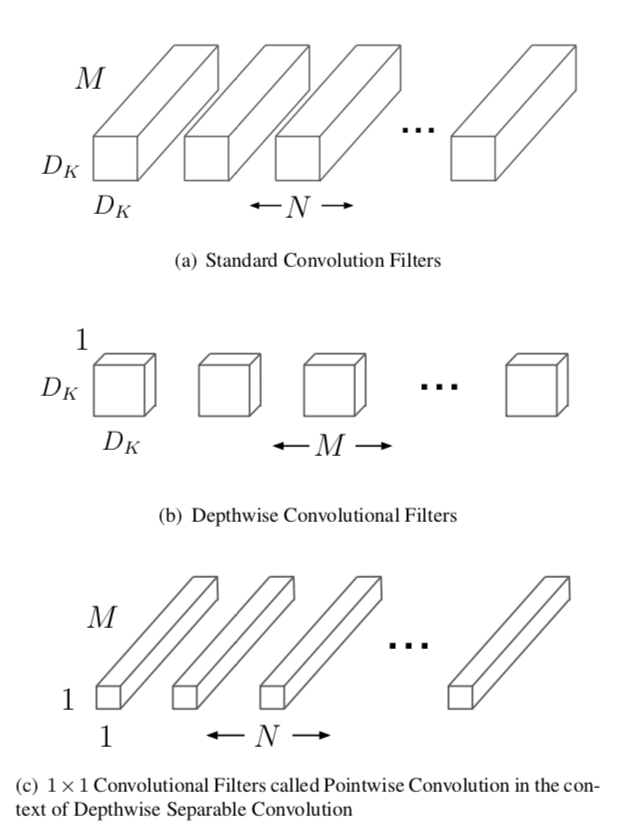图1 标准卷积与深度可分离卷积

一个标准卷积层输入DF∗DF∗M${D}_{F}\ast {D}_{F}\ast M$$D_{F}*D_{F}*M$的特征图F，并得到一个DG∗DG∗N${D}_{G}\ast {D}_{G}\ast N$$D_{G}*D_{G}*N$的输出特征图G，其中DF${D}_{F}$$D_{F}$表示输入特征图的宽和高，M是输入的通道数（输入的深度）DG${D}_{G}$$D_{G}$为输出特征图的宽和高，N是输出的通道数（输出的深度）。
标准卷积层通过由大小为DK∗DK∗M∗N${D}_{K}\ast {D}_{K}\ast M\ast N$$D_{K}*D_{K}*M*N$个卷积核K个参数，其中DK${D}_{K}$$D_{K}$是卷积核的空间维数，M是输入通道数，N是输出通道数。
标准卷积的输出的卷积图，假设步长为1，则padding由下式计算：Gk,l,n=∑i,j,mKi,j,m,n⋅Fk+i−1,l+j−1,m${G}_{k,l,n}=\sum _{i,j,m}{K}_{i,j,m,n}\cdot {F}_{k+i-1,l+j-1,m}$$G_{k,l,n}=\sum_{i,j,m}K_{i,j,m,n}{\centerdot}F_{k+i-1,l+j-1,m}$。其计算量为DK∗DK∗M∗N∗DF∗DF${D}_{K}\ast {D}_{K}\ast M\ast N\ast {D}_{F}\ast {D}_{F}$$D_{K}*D_{K}*M*N*D_{F}*D_{F}$，其由输入通道数M、输出通道数N、卷积核大小DK${D}_{K}$$D_{K}$、输出特征图大小DF${D}_{F}$$D_{F}$决定。MobileNet模型针对其进行改进。首先，使用深度可分离卷积来打破输出通道数与卷积核大小之间的相互连接作用。
标准的卷积操作基于卷积核和组合特征来对滤波特征产生效果来产生一种新的表示。滤波和组合能够通过分解卷积操作来分成两个独立的部分，这就叫做深度可分离卷积，可以大幅度降度计算成本。
深度可分离卷积由两层构成：深度卷积和逐点卷积。我们使用深度卷积来针对每一个输入通道用单个卷积核进行卷积，得到输入通道数的深度，然后运用逐点卷积，即应用一个简单的1x1卷积，来对深度卷积中的输出进行线性结合。MobileNets对每层使用batchnorm和ReLU非线性激活。
深度卷积对每个通道使用一种卷积核，可以写成：Gk,l,m^=∑i,jKi,j,m^⋅Fk+i−1,l+j−1,m$\stackrel{^}{{G}_{k,l,m}}=\sum _{i,j}\stackrel{^}{{K}_{i,j,m}}\cdot {F}_{k+i-1,l+j-1,m}$$\hat{G_{k,l,m}}=\sum_{i,j}\hat{K_{i,j,m}}{\cdot}F_{k+i-1,l+j-1,m}$，其中K̂ $\stackrel{^}{K}$$\hat{K}$是深度卷积核的尺寸DK∗DK∗M${D}_{K}\ast {D}_{K}\ast M$$D_{K}*D_{K}*M$，K̂ $\stackrel{^}{K}$$\hat{K}$中第m个卷积核应用于F中的第m个通道来产生第m个通道的卷积输出特征图Ĝ $\stackrel{^}{G}$$\hat{G}$。
深度卷积的计算量为：DK∗DK∗M∗DF∗DF${D}_{K}\ast {D}_{K}\ast M\ast {D}_{F}\ast {D}_{F}$$D_{K}*D_{K}*M*D_{F}*D_{F}$。
深度卷积相对于标准卷积十分有效，然而其只对输入通道进行卷积，没有对其进行组合来产生新的特征。因此下一层利用另外的层利用1x1卷积来对深度卷积的输出计算一个线性组合从而产生新的特征。
那么深度卷积加上1x1卷积的逐点卷积的结合就叫做深度可分离卷积，最开始在（Rigid-motion scattering for image classification.）中被提出。
深度可分离卷积的计算量为：DK∗DK∗M∗DF∗DF+M∗N∗DF∗DF${D}_{K}\ast {D}_{K}\ast M\ast {D}_{F}\ast {D}_{F}+M\ast N\ast {D}_{F}\ast {D}_{F}$$D_{K}*D_{K}*M*D_{F}*D_{F}+M*N*D_{F}*D_{F}$,即深度卷积和1x1的逐点卷积的和。
通过将卷积分为滤波和组合的过程得到对计算量的缩减：DK∗DK∗M∗DF∗DF+M∗N∗DF∗DFDK∗DK∗M∗DF∗DF=1N+1D2K$\frac{{D}_{K}\ast {D}_{K}\ast M\ast {D}_{F}\ast {D}_{F}+M\ast N\ast {D}_{F}\ast {D}_{F}}{{D}_{K}\ast {D}_{K}\ast M\ast {D}_{F}\ast {D}_{F}}=\frac{1}{N}+\frac{1}{{D}_{K}^{2}}$$\frac{D_{K}*D_{K}*M*D_{F}*D_{F}+M*N*D_{F}*D_{F}}{D_{K}*D_{K}*M*D_{F}*D_{F}}=\frac{1}{N}+\frac{1}{D^{2}_{K}}$
MobileNet使用3x3的深度可分离卷积相较于标准卷积少了8到9倍的计算量，然而只有极小的准确率的下降如第4节。
另外的空间维数的分解方式如（Flattenedconvolutional neural networks for feedforward acceleration）（Rethinking the inception architecture for computer vision.）中。但是相较于深度可分离卷积，计算量的减少也没有这么多。

3.2 网络结构和训练

MobileNet结构就像前面所提到的由深度可分离卷积所构成，且除了第一层之外为全卷积。通过用这些简单的项定义网络能够更容易的探索网络的拓扑结构来找到一个更好的网络。MobileNet结构由下表1定义。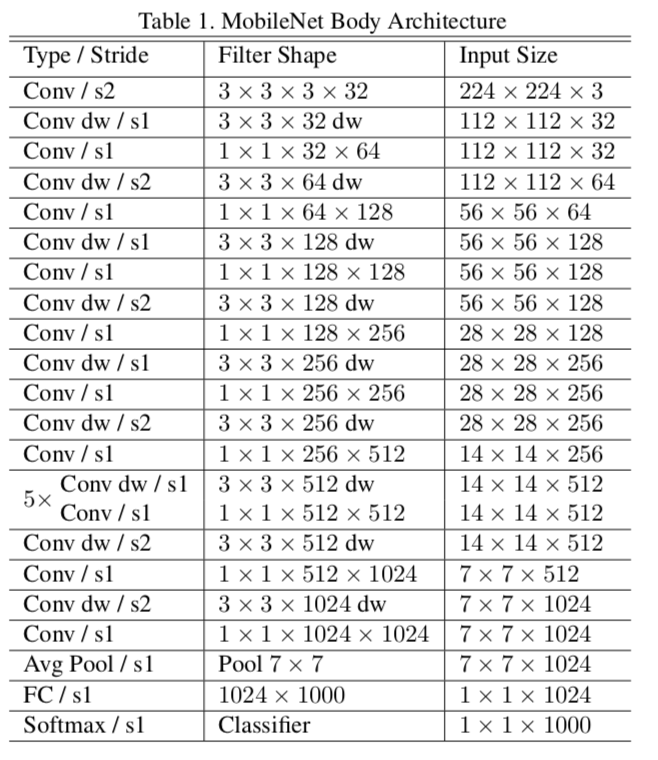表1 MobileNet的主体结构
所有的层都跟着一个batchnorm(Batch normalization: Accelerating deep network training by reducing internal covariate shift.)以及ReLU非线性激活函数，除了最后一层全连接层没有非线性激活函数直接送入softmax层进行分类。下图2比较了常规的卷积、batchnorm、ReLU层以及分解层包含深度可分离卷积、1x1卷积、以及在每层卷积层之后的batchnorm和ReLU非线性激活函数。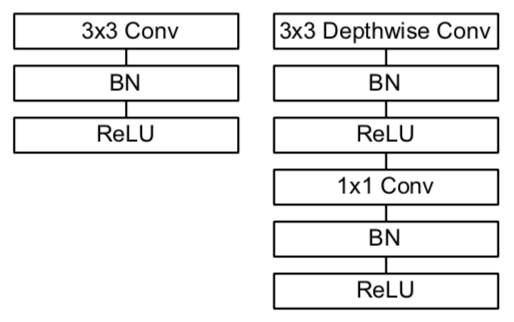图2 标准卷积与深度可分离卷积的对比
下采样通过深度可分离卷积中第一层的深度卷积通过步长来进行控制，最后将卷积层中提取到的特征图经过全局平均池化层降维至1维，然后送入全连接层分成1000类。将深度卷积和逐点卷积算作两层，则MobileNet含有28层。
用这些少数的乘加运算来定义简单的网络是不够的。确保这些操作要十分有效也是非常重要的。实例化非结构稀疏矩阵操作除非有一个非常高的稀疏度，否则不一定比稠密矩阵操作更加快速。我们的模型结构几乎将左右的计算量都放在稠密的1x1卷积中，这可以通过高度优化的GEMM通用矩阵乘法函数来实现。通常卷积由GEMM来实现，但是要求一个im2col即在内存中初始化重新排序来映射到GEMM。比如，这个方法用在caffe模型框架中（Caffe: Convolu- tional architecture for fast feature embedding）。而1x1卷积则不需要内存的重新排序，并且能直接用GEMM方法实现，因此是最优化的数值线性代数算法之一。MobileNet95%的计算时间都花费在1x1的逐点卷积上，并且占参数量的75%，如表2所示。其他额外的参数几乎都集中于全连接层。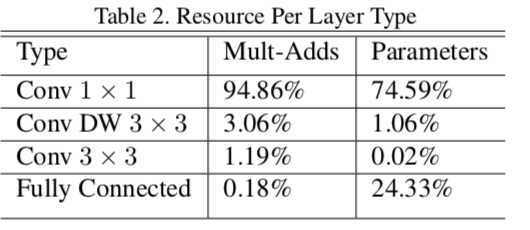MobileNet模型在Tensorflow框架(Tensorflow: Large-scale machine learning on heterogeneous systems)中使用与InceptionV3（Rethinking the inception architecture for computer vision.）中一样的RMSprop异步梯度下降算法(] T. Tieleman and G. Hinton. Lecture 6.5-rmsprop: Divide the gradient by a running average of its recent magnitude. COURSERA: Neural Networks for Machine Learning, 4(2), 2012. 4

3.3 宽度乘法器：更薄的模型

尽管最基本的MobileNet结构已经非常小并且低延迟。而很多时候特定的案例或者应用可能会要求模型变得更小更快。为了构建这些更小并且计算量更小的模型，我们引入了一种非常简单的参数α$\alpha$$\alpha$叫做宽度乘法器。宽度乘法器α$\alpha$$\alpha$的作用就是对每一层均匀薄化。给定一个层以及宽度乘法器α$\alpha$$\alpha$，输入通道数M变成了αM$\alpha M$$\alpha{M}$并且输出通道数变成αN$\alpha N$$\alpha{N}$。
加上宽度乘法器的深度可分离卷积的计算量如下：DK∗DK∗αM∗DF∗DF+αM∗αN∗DF∗DF${D}_{K}\ast {D}_{K}\ast \alpha M\ast {D}_{F}\ast {D}_{F}+\alpha M\ast \alpha N\ast {D}_{F}\ast {D}_{F}$$D_{K}*D_{K}*\alpha{M}*D_{F}*D_{F}+\alpha{M}*\alpha{N}*D_{F}*D_{F}$
由于α∈(0,1]$\alpha \in \left(0,1\right]$$\alpha{\in}(0,1]$，一般设置为1\0.75\0.5\0.25。当α=1$\alpha =1$$\alpha=1$的时候就是最基本的MobileNet，当α<1$\alpha <1$$\alpha<1$时，就是薄化的MobileNet。宽度乘法器对计算量和参数量的减少大约α2${\alpha }^{2}$$\alpha^{2}$倍。宽度乘法器可以应用在任何模型结构来定义一个更瘦的模型，并且权衡合理的精度、延迟的大小。宽度乘法器常用来薄化一个新的需要从头开始训练的网络结构。

3.4 分辨率乘法器：约化表达

第二个薄化神经网络计算量的超参数是分辨率乘法器ρ$\rho$$\rho$。我们将其应用在输入图片以及每一层的内部表达中。实际上，我们通过设置ρ$\rho$$\rho$来隐式的设置输入的分辨率大小。
我们现在可以对网络中的核心层的深度可分离卷积加上宽度乘法器α$\alpha$$\alpha$以及分辨率乘法器ρ$\rho$$\rho$来表达计算量：DK∗DK∗αM∗ρDF∗ρDF+αM∗αN∗ρDF∗ρDF${D}_{K}\ast {D}_{K}\ast \alpha M\ast \rho {D}_{F}\ast \rho {D}_{F}+\alpha M\ast \alpha N\ast \rho {D}_{F}\ast \rho {D}_{F}$$D_{K}*D_{K}*\alpha{M}*\rho{D_{F}*\rho{D_{F}}}+\alpha{M}*\alpha{N}*\rho{D_{F}*\rho{D_{F}}}$
其中ρ∈(0,1]$\rho \in \left(0,1\right]$$\rho{\in}(0,1]$，一般隐式的设置以便于输入网络的图像分辨率为224\192\160\128等。当ρ=1$\rho =1$$\rho=1$时为最基本的MobileNet，当ρ<1$\rho <1$$\rho<1$时，则为薄化的MobileNet。分辨率乘法器对网络约化大约ρ2${\rho }^{2}$$\rho^{2}$倍。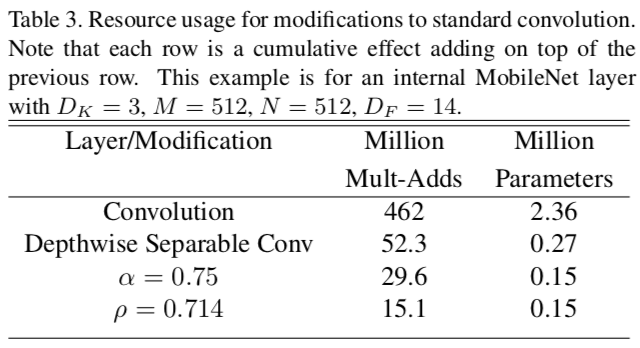4 实验

在这一节，我们首先调研了深度可分离卷积以及通过收缩网络的宽度而不是减少网络的层数带来的影响。然后展示了基于两个超参数：宽度乘法器和分辨率乘法器，来收缩网络的权衡，并且与一些著名的网络模型进行了对比。然后调研了MobileNet运用在一些不同的应用上的效果。

4.1 模型选择

首先我们展示了运用深度可分离卷积的MobileNet与全标准卷积网络的对比，如表4，我们可以看见在ImageNet数据集上使用深度可分离卷积相较于标准卷积准确率只减少了1%，但在计算量和参数量上却减少了很多。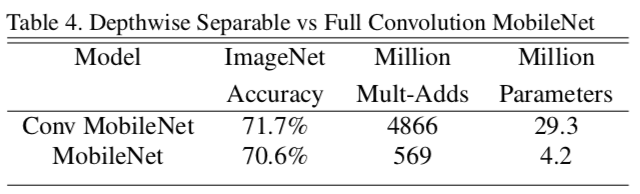接下来，我们展示了利用宽度是乘法器的薄化模型与只有少数层的千层神经网络进行对比，为了使MobileNet更浅，表1中的5层14x14x512的特征尺寸的可分离卷积层都被去掉了。表5展示了相同计算量参数量的情况下，让MobileNets薄化3%比让它更浅效果更好。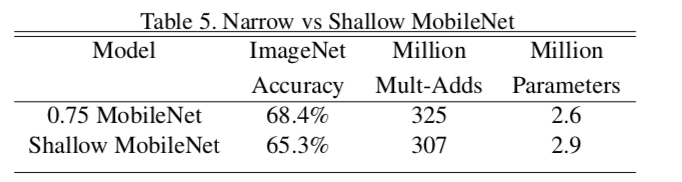4.2 模型压缩超参数

表6展示了利用宽度乘法器α$\alpha$$\alpha$对MobileNet网络结构进行薄化后准确率，计算量和尺寸之间的权衡关系。准确率直到宽度乘法器α$\alpha$$\alpha$下降到0.25才显示下降很多。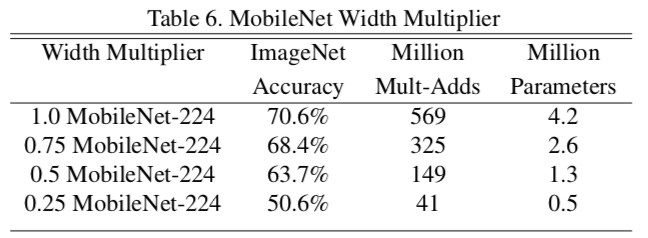表7展示了通过利用约化的MobileNets时不同分辨率乘法器时准确率、计算量和尺寸之间的权衡关系。准确率随着分辨率下降而平滑减小。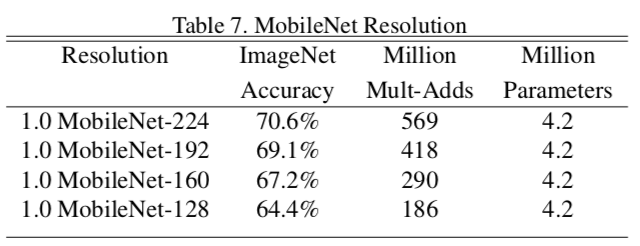图3显示了16个不同的模型在ImageNet中准确率和计算量之间的权衡。这16个模型由4个不同的宽度乘法器{1,0.75,0.5,0.25}以及不同分辨率{224,192,160,128}组成。当α$\alpha$$\alpha$=0.25时，模型变得非常小，整个结果呈现对数线性跳跃。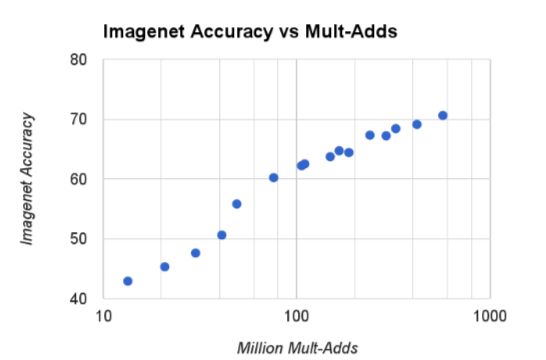图3
图4显示了16个不同模型在ImageNet中准确率和参数量之间的权衡。这16个模型由4个不同的宽度乘法器{1,0.75,0.5,0.25}以及不同分辨率{224,192,160,128}组成。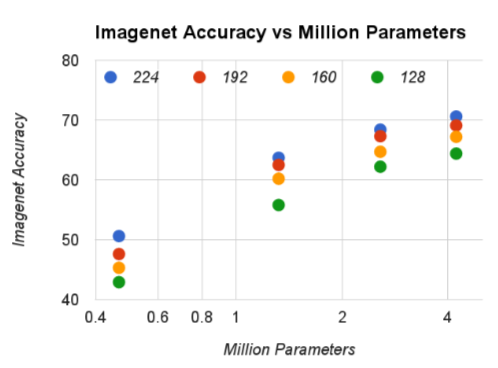图4 颜色编码输入的分辨率，参数的数量不随输入分辨率而变化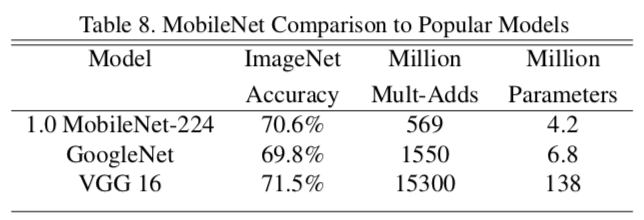表9比较了约化后的MobileNet(α=0.5$\alpha =0.5$$\alpha=0.5$，并且分辨率为160x160，原本为224x224)与AlexNet以及SqueezeNet( Squeezenet: Alexnet-level accuracy with 50x fewer parameters and¡ 1mb model size. )。约化后的MobileNet相较于这两个模型，准确率都高，并且计算量相较于AlexNet少了9.4倍比SqueezeNet少了22倍。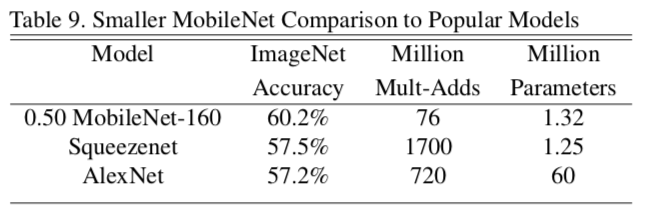4.3 细粒度识别

在斯坦福狗数据集（Novel dataset for fine-grained image categorization）上训练MobileNet来进行细粒度识别。我们扩展了（The unreasonable effectiveness of noisy data for fine-grained recognition.）中的方法，并且从网上收集了一个相对其更大，噪声更多的训练集，我们使用网上的噪声数据集先预训练一个细粒度识别狗的模型，然后在斯坦福狗数据集上进行精调。结果显示在表10中。MobileNet几乎可以实现最好的结果，并且大大减少了计算量和尺寸。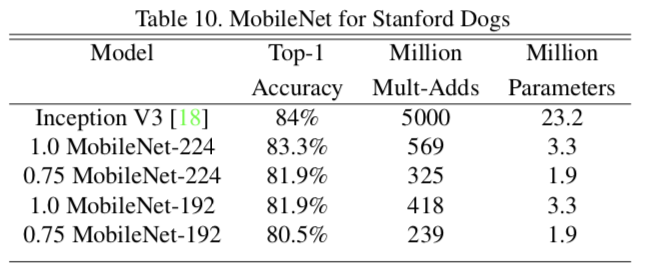4.4 大规模地理信息

（PlaNet - Photo Geolocation with Convolutional Neural Networks）描述了在哪里拍照是一个分类问题。这个方法将地球分割为地理网格作为目标类来利用数以百万的地理标记图片训练一个卷积神经网络。PlaNet已经成功定位了大量照片，相对（ IM2GPS: estimating geographic in- formation from a single image）（Large-Scale Image Geolocalization.）针对相同的任务，效果更好。
我们在相同数据集上利用MobileNet结构重新训练PlaNet，因为PlaNet基于inceptionV3结构，其有5200万参数以及5.74亿的乘加计算量。而MobileNet只有1300万参数量以及几百万的乘加计算量。在表11中，MobileNet相较于PlaNet只有少数准确率的下降，然而大幅度的优于Im2GPS。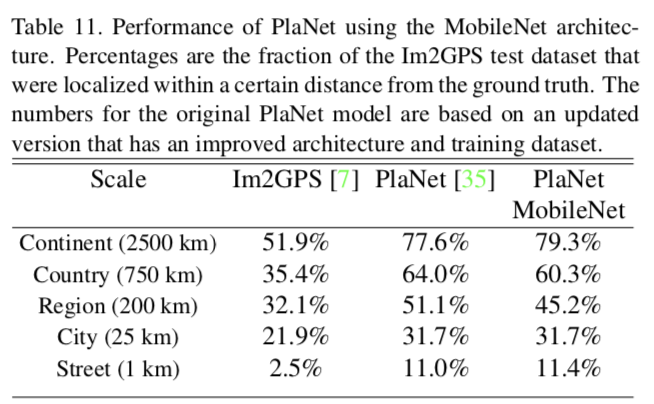4.5 人脸属性

另一个MobileNet的使用实例就是利用未知深奥的训练过程来压缩大型系统。在人脸属性分类任务中，我们证明了MobileNet与蒸馏（一种针对深层网络的知识转换理论）（Distilling the knowledge in a neural network）之间的协同关系。我们利用7500万参数以及16亿乘加运算计算量来约化一个大型人脸属性分类器。这个分类器在一个类似于YFCC100M数据集（Yfcc100m: The new data in multimedia research.）上的一个多属性数据集上训练。
我们使用MobileNet结构提炼一个人脸属性分类器。通过训练分类器来蒸馏工作来模拟一个大型模型的输出，而不是真实的标签。因此能够训练非常大（接近无限）的未标记的数据集。结合蒸馏训练的可扩展性以及MobileNet的简约参数化，终端系统不仅要求正则化（权重衰减和早停），而且增强了性能。如表12中可以明显看到基于MobileNet-base分类器针对模型收缩更有弹性变化：它在跨属性间实现了一个相同的mAP但是只用了呀哪里1%的乘加运算。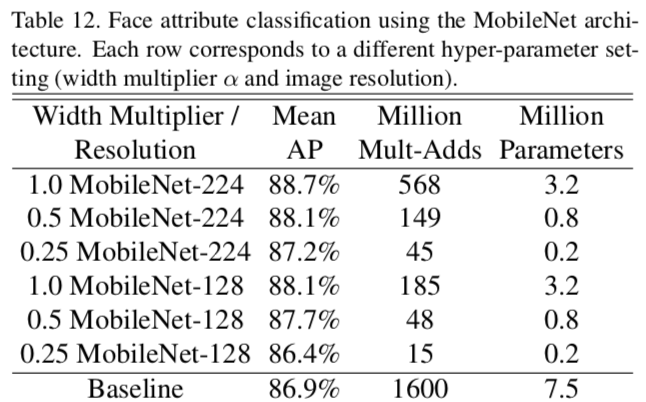4.6 目标检测

MobileNet也可以作为一个基本网络部署在现代目标检测系统中。我们在COCO数据集上训练得到结果并且赢得了2016COCO挑战赛。在表13中，MobileNet与VGG和InceptionV2在Faster-RCNN以及SSD(Ssd: Single shot multibox detector.)框架下进行比较。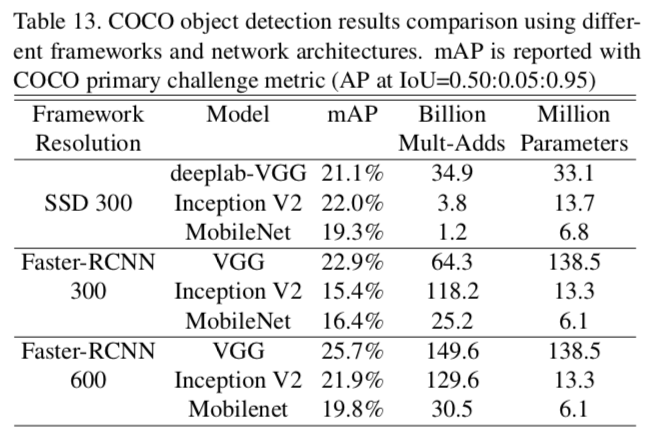在我们的实验中，SSD由分辨率为300的输入图片进行检测，Faster-RCNN有300和600两种分辨率进行比较。Faster-RCNN模型每张图片测试了300RPN候选区域框，模型利用COCO的训练和验证集进行训练，包含了8000张微缩图片，并且在微缩图片中进行测试。对于上述框架，MobileNet与其他网络进行比较，计算复杂度和模型尺寸相当于其他模型的一小部分。

4.7 人脸嵌入

FaceNet是艺术人脸识别模型中最好的（Facenet: A uni- fied embedding for face recognition and clustering.）它构建了基于三次损失的人脸嵌入。为了构建移动端FaceNet模型，我们在训练集上通过最小化FaceNet和MobileNet之间的平方差来蒸馏训练。结果展示在表14中。5 结论

我们提出了一个新的模型基于深度可分离卷积网络结构MobileNet。我们调研了一些重要的设计决策来引领一个有效的模型。然后我们描述了如何使用宽度乘法器和分辨率乘法器通过权衡准确率来减少尺寸和延迟来构建更小更快的MobileNets。然后将MobileNet与著名的模型在尺寸、速度和准确率上进行比较。我们总结了当MobileNet应用在各种任务中的有效性。下一步为了帮助探索MobileNets的更多改进和应用，我们计划在tensorflow中加入MobileNet。
展开全文卷积神经网络 模型压缩
• ## MobileNet v1 和 v2

千次阅读 2020-03-03 21:09:14
1 MobileNet V1 MobileNet是针对移动端优化的卷积，所以当需要压缩模型时，可以考虑使用MobileNet替换卷积。下面我们开始学习MobileNet原理，并且先通过Tensorflow函数接口实现MobileNet，再手写python代码实现...
1 MobileNet V1

MobileNet是针对移动端优化的卷积，所以当需要压缩模型时，可以考虑使用MobileNet替换卷积。下面我们开始学习MobileNet原理，并且先通过Tensorflow函数接口实现MobileNet，再手写python代码实现MobileNet。

1.1 普通卷积

MobileNet是用于替换普通卷积，相比普通卷积，MobileNet参数更少，计算速度更快。我们先看一下输入为(h=12,w=12,c=4)，卷积为3*3，输出为（h=12,w=12,c=2）前向计算中，普通卷积的参数量、乘法计算次数。普通卷积如下图所示：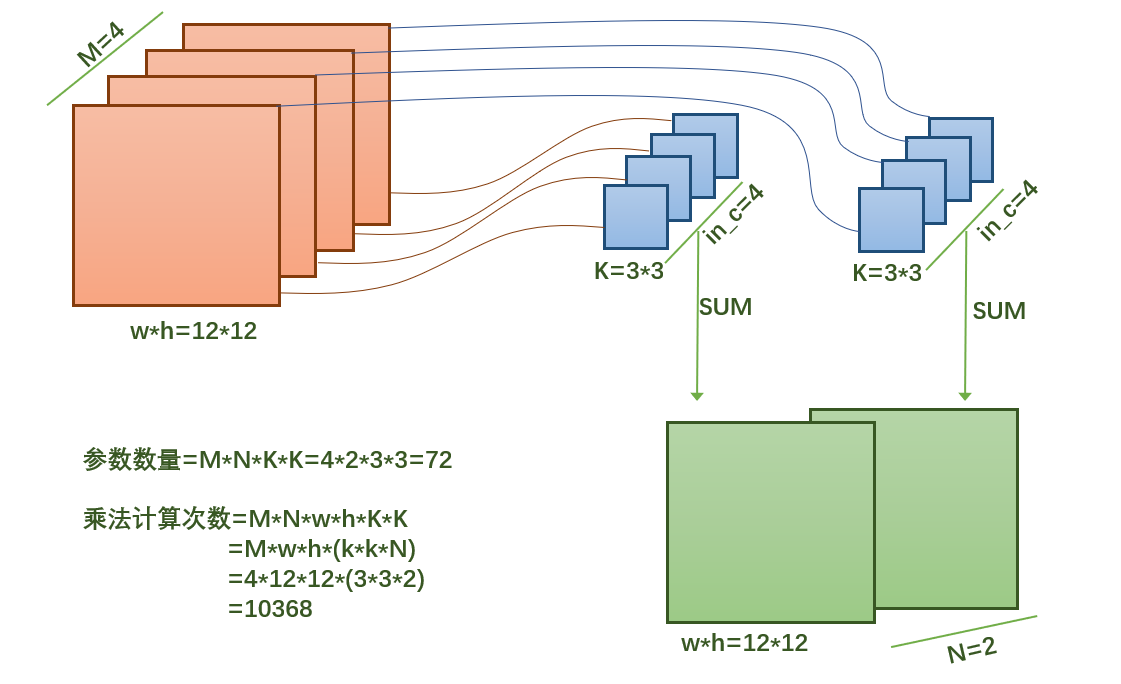从上图可以很简单的计算到，普通卷积参数总数为72个，需要做10368次乘法计算。

1.2 MobileNet v1

相比普通卷积，MobileNet采用的方法是，将卷积分解为2个操作：depthwise和pointwise。pointwise比较容易理解，就是普通的卷积核为1*1的卷积。depthwise采用的方法不是普通卷积方式，我们知道，对于输入通道数为4的feature map在计算卷积时，输出的每个通道都需要对应4个3*3卷积核参数。这一步是最主要的耗时，为了提升计算速度，MobileNet把每个输入feature map对应一个3*3卷积核，输出通道数不变，即为4。而真正对通道数做改变的是在pointwise，也就是1*1的卷积。

下面图很清晰的理解mobilenet原理：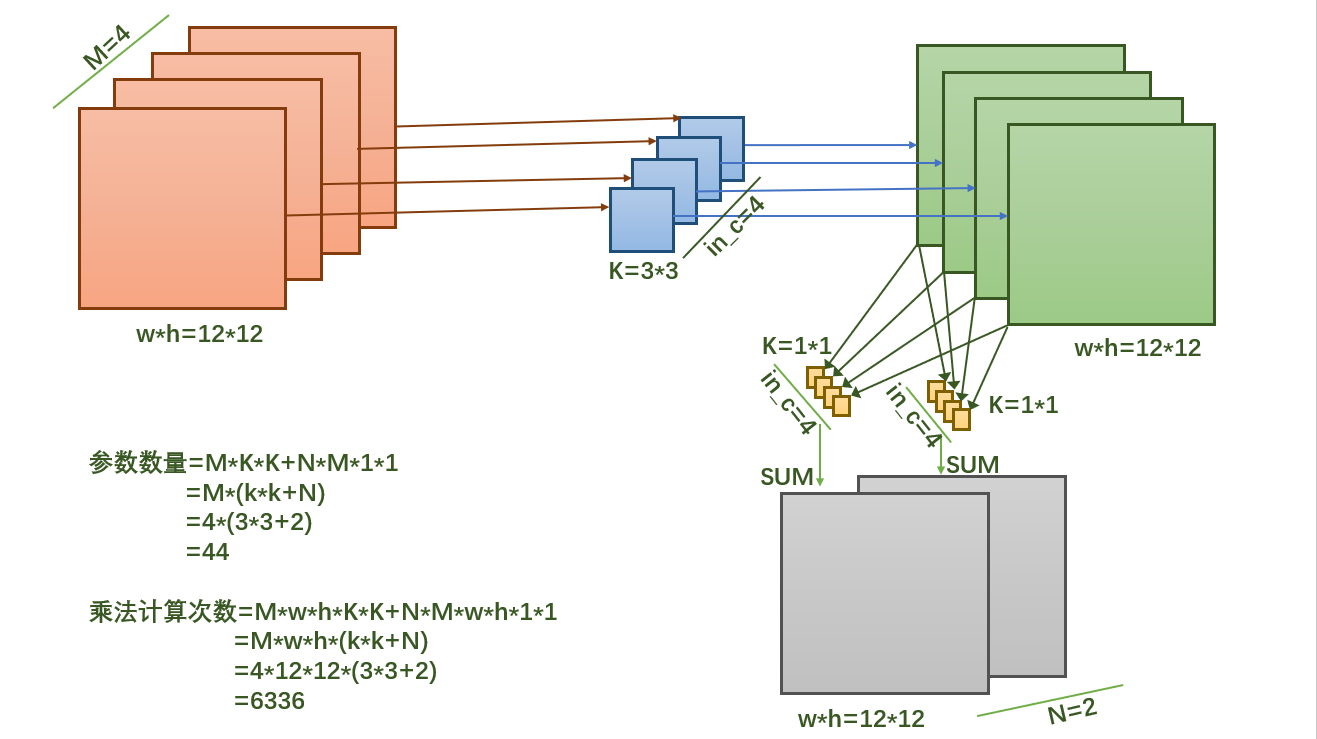从上图可以很简单的计算到，普通卷积参数总数为44个，需要做6336次乘法计算。可以看到，mobilenet的参数和乘法计算次数明显比普通卷积要小。这还仅仅是我列举的简单例子，在实际网络中，几十层的网络很常见，feature map也是远远大于12*12*4。根据我的经验，普通100M的网络模型，将所有卷积替换成mobilenet后，能降到20M以下，计算速度更是不在一个量级。

Mobilenet v2

主要贡献

有2点

1，提出了逆向的残差结构（Inverted residuals）

由于MobileNetV2版本使用了残差结构，和resnet的残差结构有异曲同工之妙，源于resnet，却和而不同。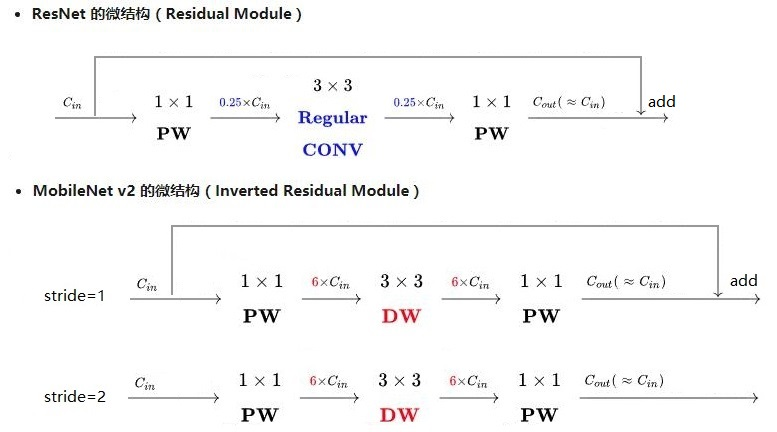由于Resnet没有使用depthwise conv,所以，在进入pointwise conv之前的特征通道数是比较多的，所以，残差模块中使用了0.25倍的降维。而MobileNet v2由于有depthwise conv，通道数相对较少，所以残差中使用 了6倍的升维。

总结起来，2点区别

（1）ResNet的残差结构是0.25倍降维，MobileNet V2残差结构是6倍升维

（2）ResNet的残差结构中3*3卷积为普通卷积，MobileNet V2中3*3卷积为depthwise conv

MobileNet v1，MobileNet v2 的区别

有2点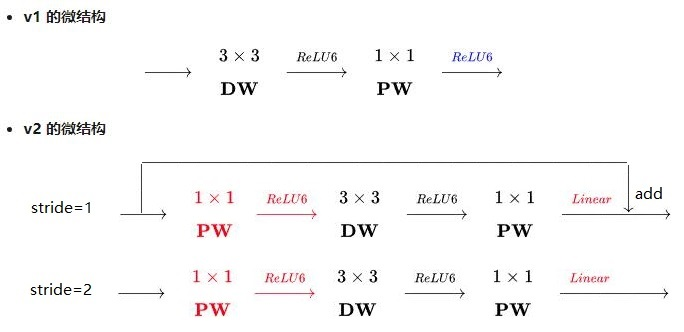（1）v2版本在进入3*3卷积之前，先进行了1*1pointwise conv升维，并且经过RELU。

（2）1*1卷积出去后，没有进行RELU操作

MobileNet v1，MobileNet v2 的实际使用区别：

精度上，MobileNet v2优于MobileNet v1

速度上，在GPU有并行的情况下，v1和v2速度基本差不多，在cpu这种无并行的情况下，v2要比v1慢大概40%。就是说，v2比v1的乘加运算量确实是小，但是最终速度是由好多因素决定的，这也就是shuffle v2的改进由来。

MobileNet v1，MobileNet v2 的实际使用区别：

精度上，MobileNet v2优于MobileNet v1

速度上，在GPU有并行的情况下，v1和v2速度基本差不多，在cpu这种无并行的情况下，v2要比v1慢大概40%。就是说，v2比v1的乘加运算量确实是小，但是最终速度是由好多因素决定的，这也就是shuffle v2的改进由来。

2，提出了线性瓶颈单元（linear bottlenecks）

Why no RELU？

首选看看RELU的功能。RELU可以将负值全部映射为0，具有高度非线性。下图为论文的测试。在维度比较低2,3的时候，使用RELU对信息的损失是比较严重的。而单维度比较高15,30时，信息的损失是比较少的。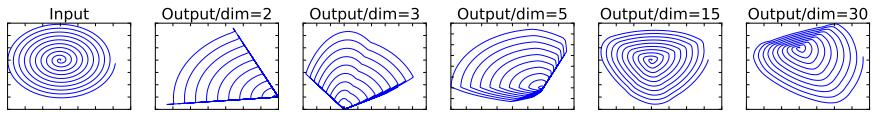MobileNet v2中为了保证信息不被大量损失，应此在残差模块中去掉最后一个的RELU。因此，也称为线性模块单元。

MobileNet v2网络结构：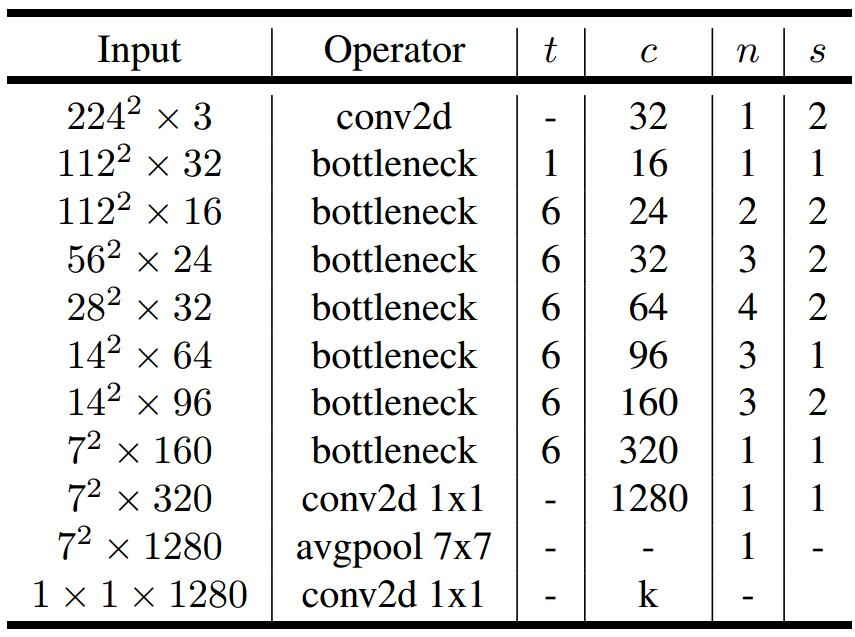其中，t表示通道的扩大系数expansion factor，c表示输出通道数，

n表示该单元重复次数，s表示滑动步长stride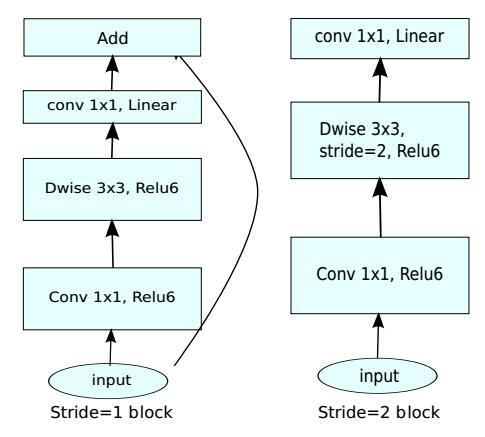其中bottleneck模块中，stride=1和stride=2的模块分别如上图所示，只有stride=1的模块才有残差结构。

结果：

MobileNet v2速度和准确性都优于MobileNet v1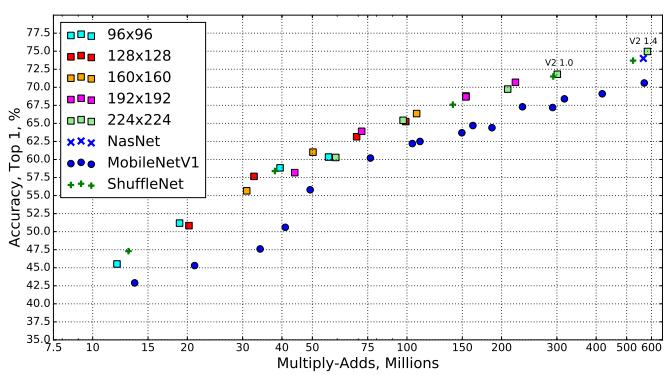和其他结构的对比：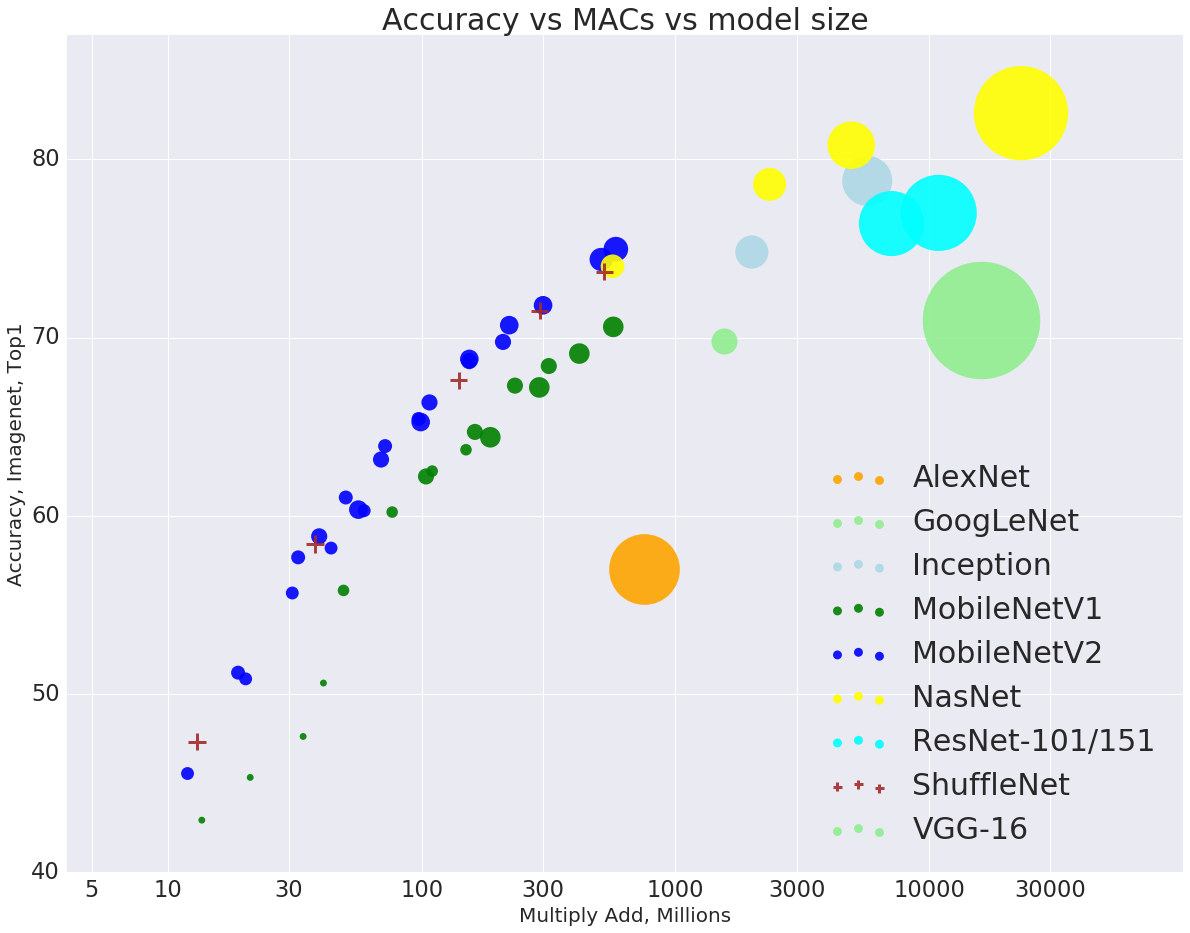相关论文

相关中文论文
邢艳芳，卓文鑫，段红秀. 基于MobileNet的敏感图像识别系统设计[J]. 电视技术, 2018, 42(7):53-56.
童星，张激. 基于SSD_MobileNet模型的ROS平台目标检测[J]. 计算机系统应用, 2019, 28(1):94-99.
曹香滢，孙卫民，朱悠翔 . 基于科优先策略的植物图像识别[J]. 计算机应用, 2018, 38(11):195-199.
黄宁波. 用于目标检测的深度学习算法优化研究[D]. 长春理工大学, 2018.
杨洁.基于卷积神经网络的目标检测研究[D]. 南华大学, 2018.

引用：

https://blog.csdn.net/qq_14845119/article/details/73648100

https://blog.csdn.net/huachao1001/article/details/79171447

展开全文• 手机号和省市对照表.txt文件格式，没有区域码，只有省市，如:1300000,山东:济南 1300001,江苏:常州 1300002,安徽:巢湖
• ## MobileNetV1 & MobileNetV2 简介

万次阅读 多人点赞 2020-07-10 15:24:39
一、参数数量和理论计算量 1、定义 参数数量（params）：关系到模型大小，单位通常为M，通常参数用 float32 表示，所以模型大小是参数数量的 4 倍 理论计算量（FLOPs）： ...是 floating point operations 的...
• discuz论坛多端应用源码之后端插件 使用discuz论坛作为后端生成微信、QQ、支付宝、头条抖音及百度小程序，并支持安卓或苹果APP.
• mobileSelect.js一款多功能的手机移动端选择组件，支持单选到多选、支持多级级联、提供自定义回调函数、提供update函数二次渲染、重定位函数、兼容pc端拖拽等等。mobileSelect.js是一个js移动端选择控件，不依赖任何...手机选择插件 js移动端选择插件
• 我对UI框架的一些想法最近一年多一直在做UI库，从 SUI Mobile -> Light7 -> jQuery WeUI。自己也在做的过程中对UI库类的产品有了更深入的认识，SUI Mobile 和 Light7 有一个很大的问题在于：试图用一个轻量的基于...VUI Vue Vue-ui
• ## antd-mobile的listView踩坑笔记

万次阅读 热门讨论 2019-08-31 12:08:23listView
• 这段时间一直在忙着做手机版和微信版预约，由于公司没有专业的美工，所以就在网上找了手机端的前端框架jquerymobile，由于公司没有jquerymobile的使用经验，基本上是边开发边学习，用到的效果现查先用。但是在实际...
• WIN10下windows mobile设备中心连接不上 网上的教程都是把基于Windows mobile2003的设备连接里的登录身份改为本地系统账户。 这个必须是要之前设备成功连接上电脑改了才会有效，不然会发现还是连不上。 其实还要把...
• 1.本文档只做为E-mobile4.5服务器端安装配置手册！ 2.E-Mobile4.5可运行在windows和linux服务器上 3.E-Mobile4.5必须与ecology服务器互联，因此， Ø 请确保在E-Mobile所在的服务器上可以通过浏览器访问Ecology...
• ## jquerymobile设计完整例子

千次下载 热门讨论 2020-07-30 23:32:24
jquerymobile例子 包含从登陆到菜单和各种菜单切换完整设计 上传只为分享
• jQuery Mobile修改button的内容。 使用jQuery Mobile也有一段时间了，最近同事提出，他需要更改button的内容，但是修改不了。我也试着修改了几次，button的内容其实是更改了，但是页面并未刷新。在调试中使用了...html5 button禁用 button隐藏
• 一直在用ant.design桌面版，现在退出了移动版，必须研究一下，但是这不看不要紧，一看就遇到了好多坑，好多坑···1....\$ dva new mobileApp创建完成后，已经是npm install过了，所以不需要重新下载依赖。框架 结构 ant-mobile
• windows键+R打开【运行】（或者你手动查找），输入services.msc，在服务上找到这两个服务：Windows Mobile 的设备 和 基于 Windows Mobile 2003 的设备连接，右键这两个服务，启动类型原来的 自动（延迟启动）或...同步软件
• 滑动手势在移动设备是很流行的，在移动设备中滑动翻页中很常见 虽然这个功能可以在jQueryMobile中实现，但是个人与之前写的《【jQuery】论手机浏览器中拖拽动作的艰难性》（点击...那么，使用jQueryMobile实现滑动翻页手机浏览器 web前端 jquery
• jQuery.mobile.changePage方法用的还是很多的。作为一个老手，有必要对jQuery mobile中实用方法做一些总结。系列文章请看jQuery Mobile专栏。jquery.mobile.changepage是过时的jQuery Mobile 1.4.0及将被删除在1.5.0...html5
• jQuery Mobile使用指南 简介：jQuery Mobile框架可以轻松的帮助我们实现非常好看的、可跨设备的Web应用程序。我们将后续的介绍中向大家介绍大量的代码及实例。 jQuery一直以来都是非常流行的富客户端及Web...jquery mobile stylesheet div listview
...